Nielsen–Ninomiya theorem

Known as: Nielsen-Ninomiya theorem
The Nielsen–Ninomiya theorem is a no-go theorem in physics, in particular in lattice gauge theory, concerning the possibility of defining a theory of… Expand
Wikipedia

Papers overview

Semantic Scholar uses AI to extract papers important to this topic.
2017
2017
The Nielsen-Ninomiya theorem implies that any local, Hermitian and translationally invariant lattice action in even-dimensional… Expand
•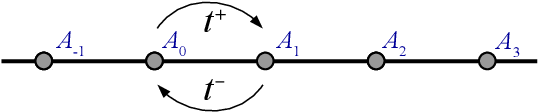•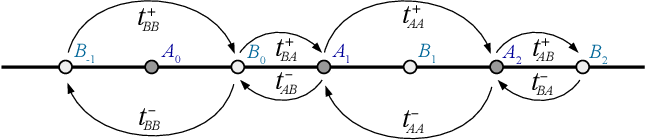•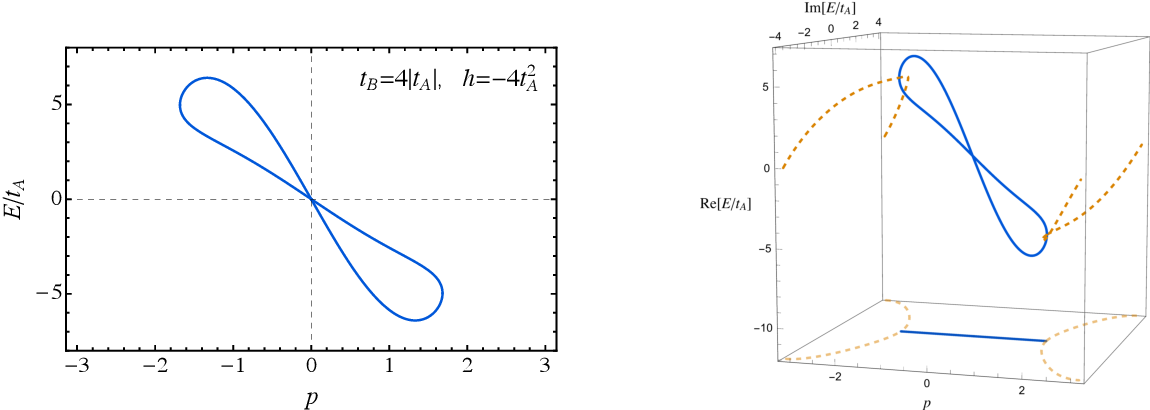•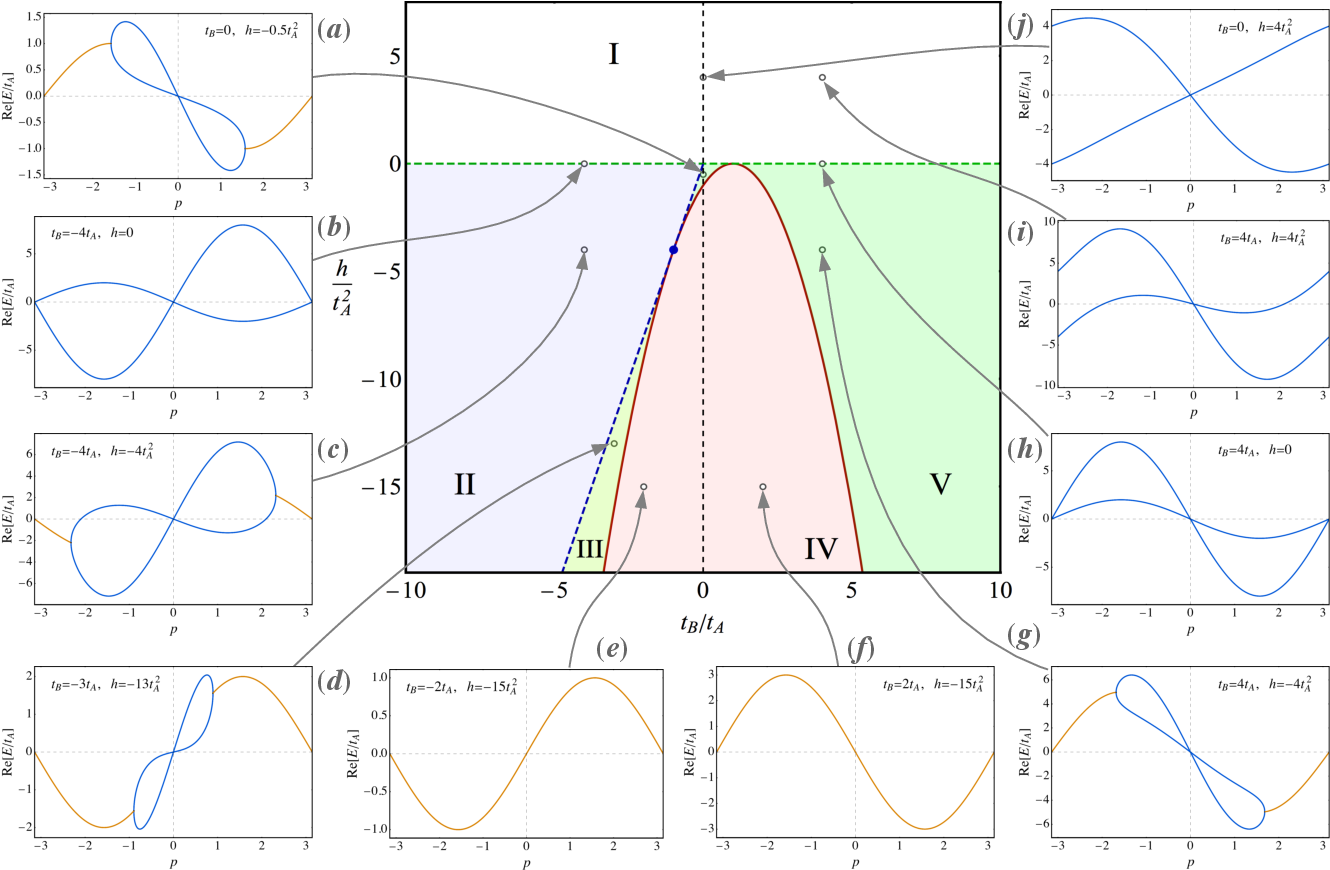•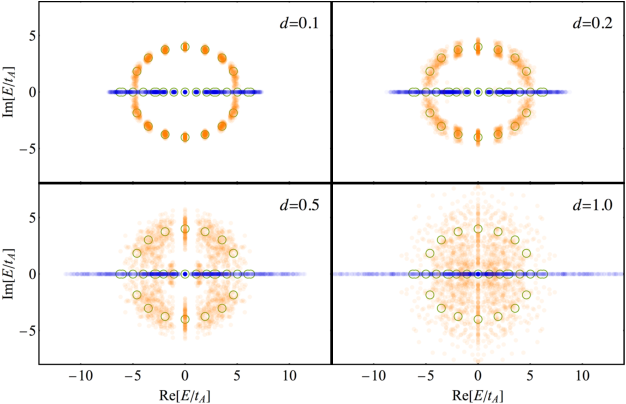Is this relevant?
2015
2015
The same leptoquarks that explain the recently observed anomaly in $R_K$ can generate naturally small Majorana neutrino masses at… Expand
•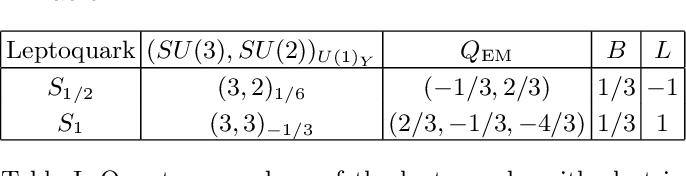•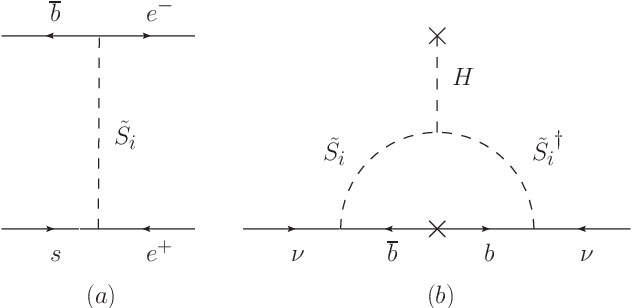•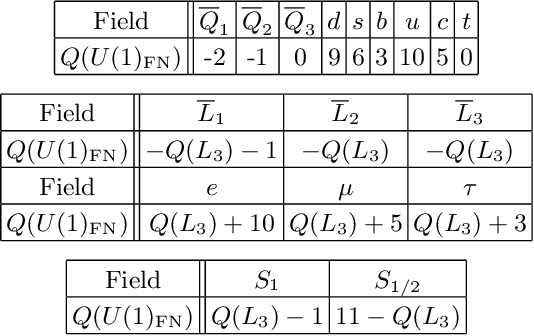Is this relevant?
2013
2013
Let $${(\phi, \psi)}$$(ϕ,ψ) be an (m, n)-valued pair of maps $${\phi, \psi : X \multimap Y}$$ϕ,ψ:X⊸Y, where $${\phi}$$ϕ is an m… Expand
Is this relevant?
2011
2011
Numerous empirical studies have shown that certain exponential Levy models are able to fit the empirical distribution of daily… Expand
•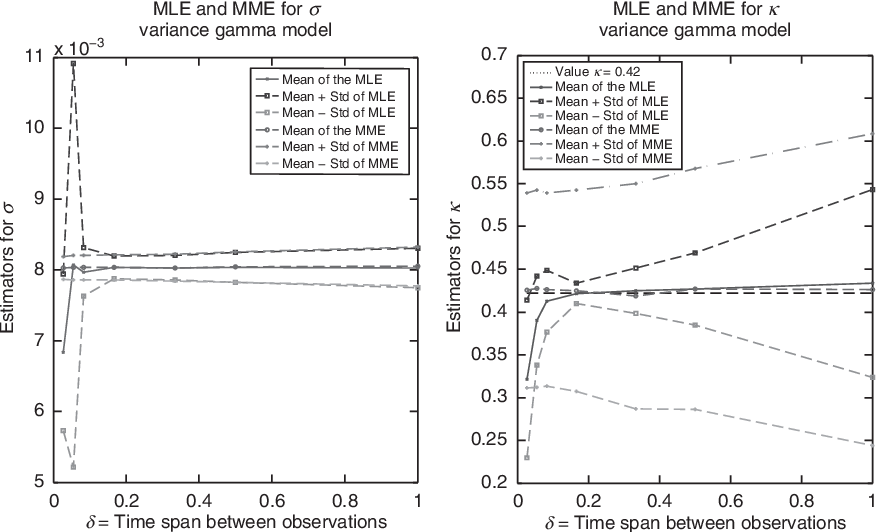•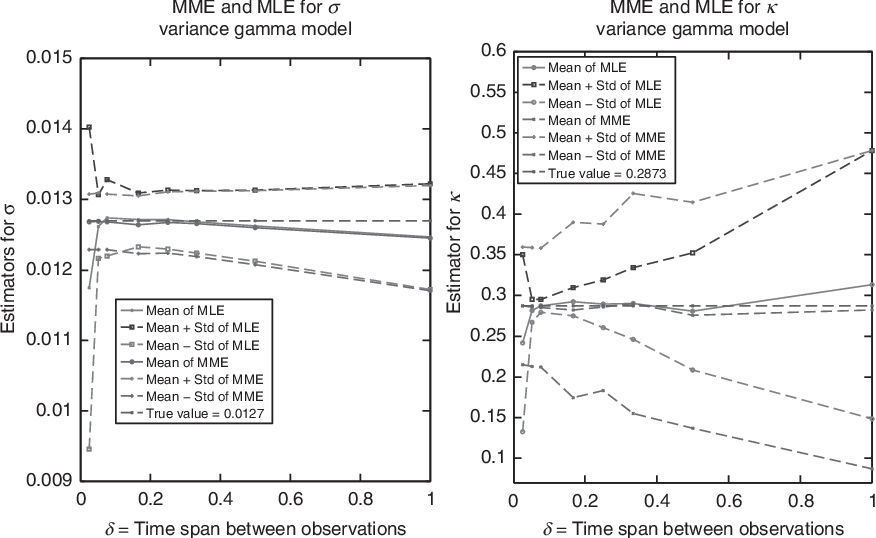•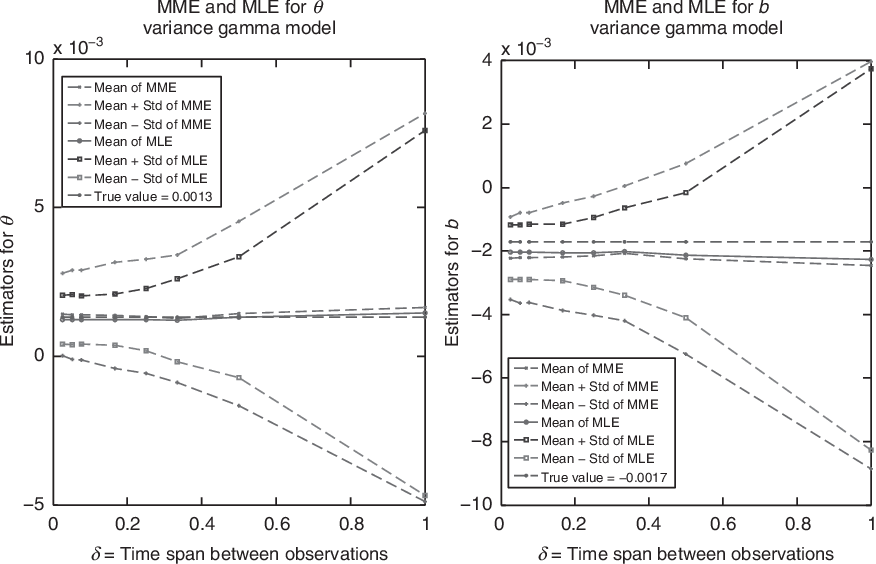•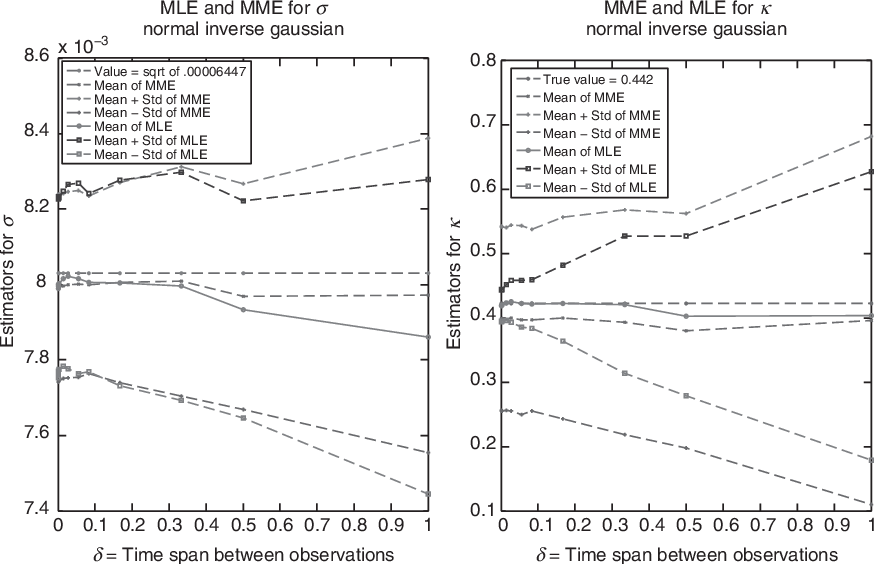•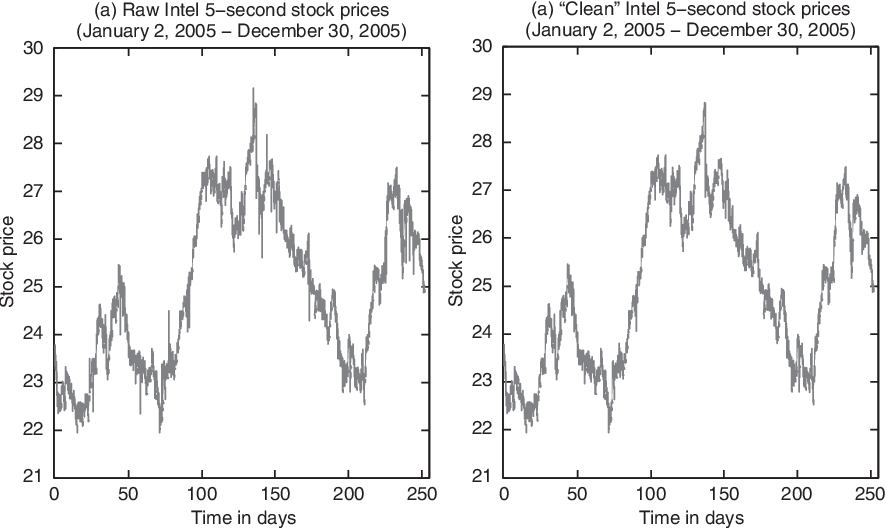Is this relevant?
2009
2009
Among the soil formation factors, relief is one of the most used in soil mapping, because of its strong correlation with the… Expand
•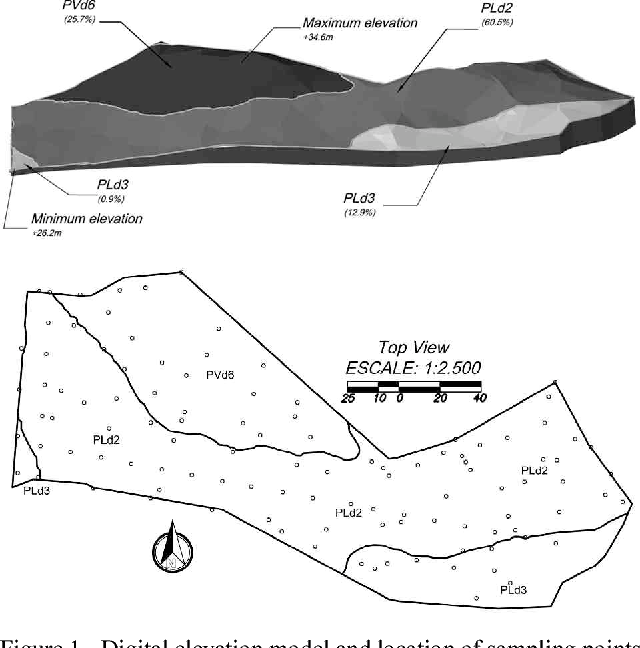•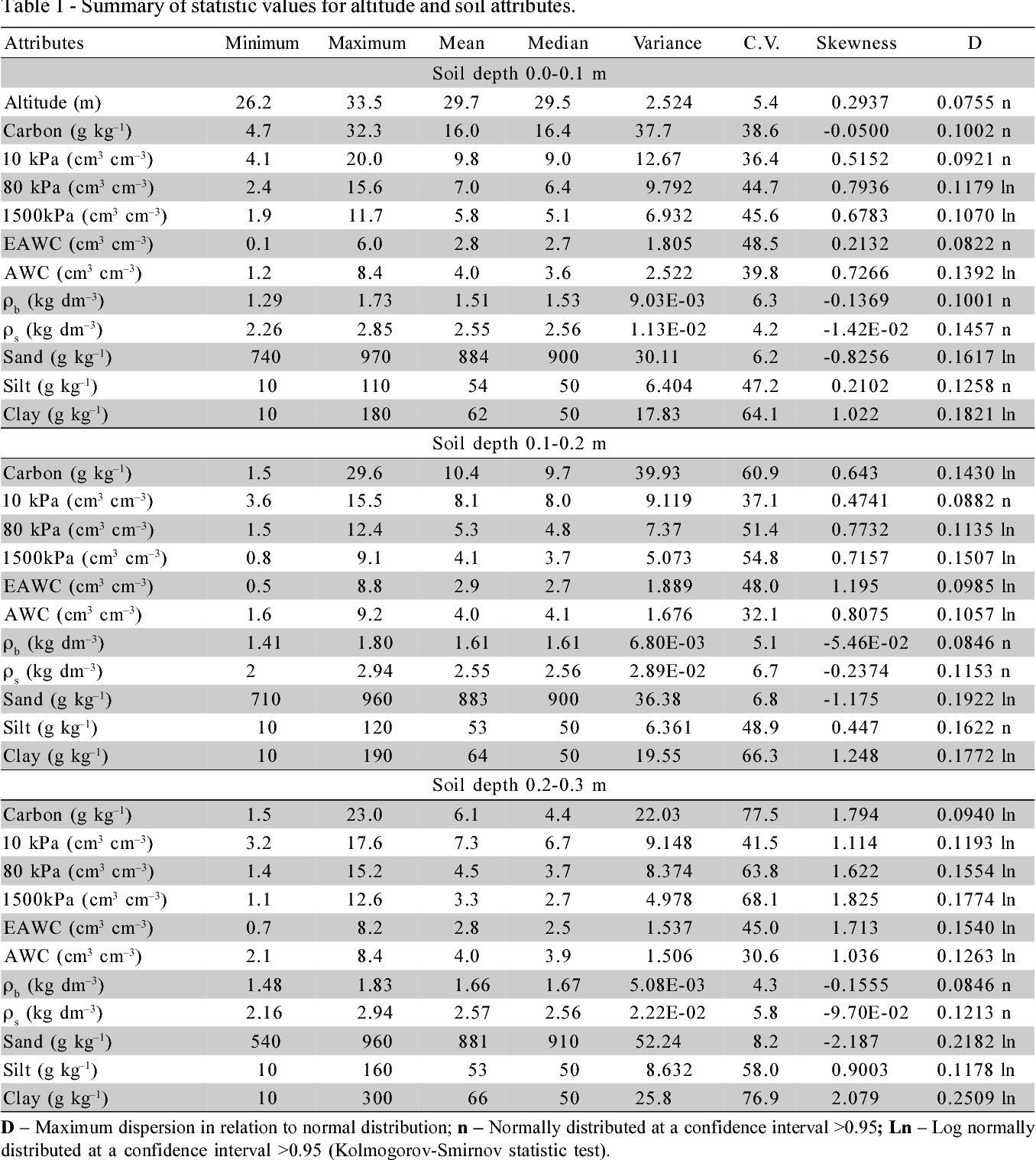•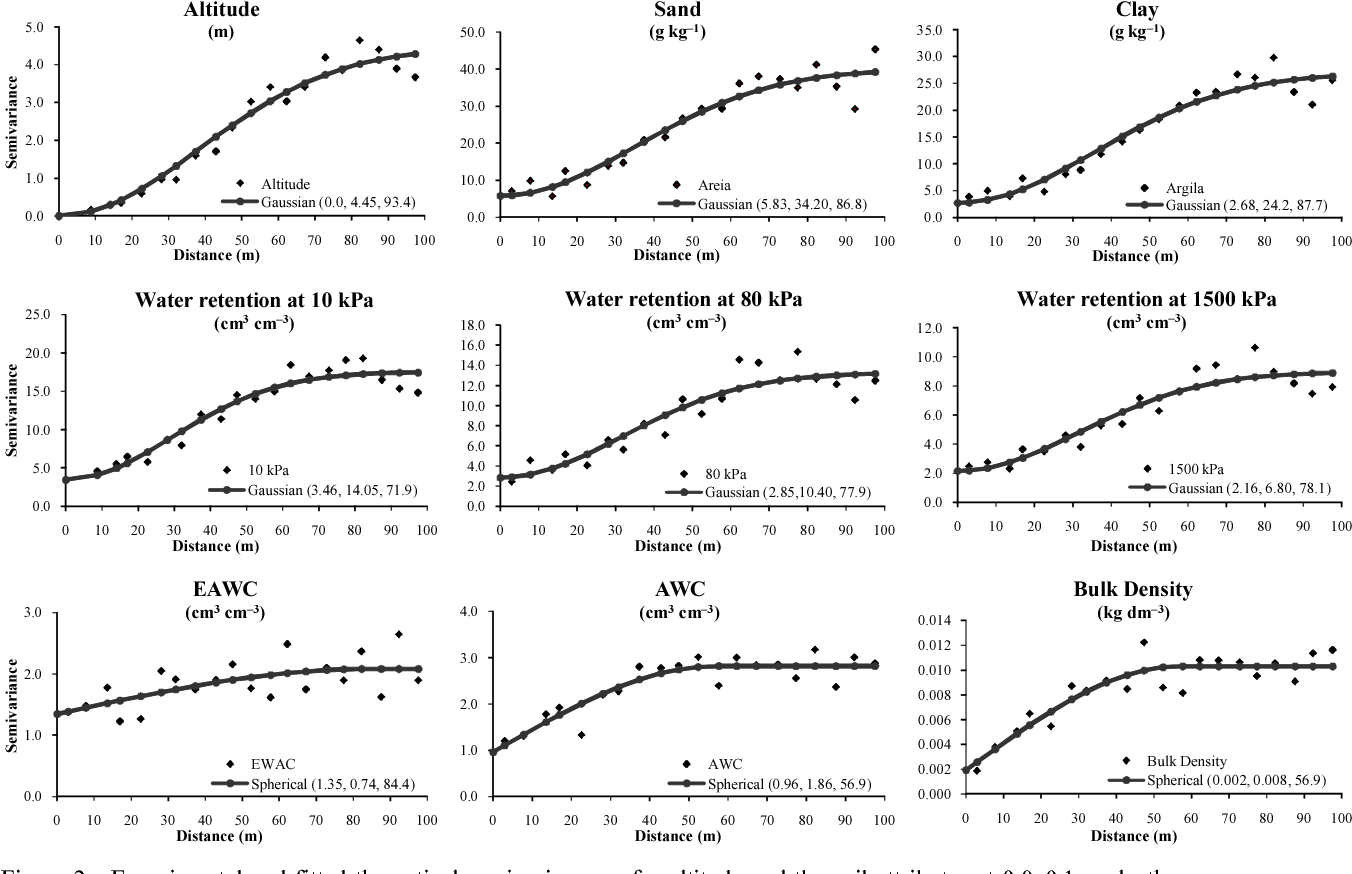•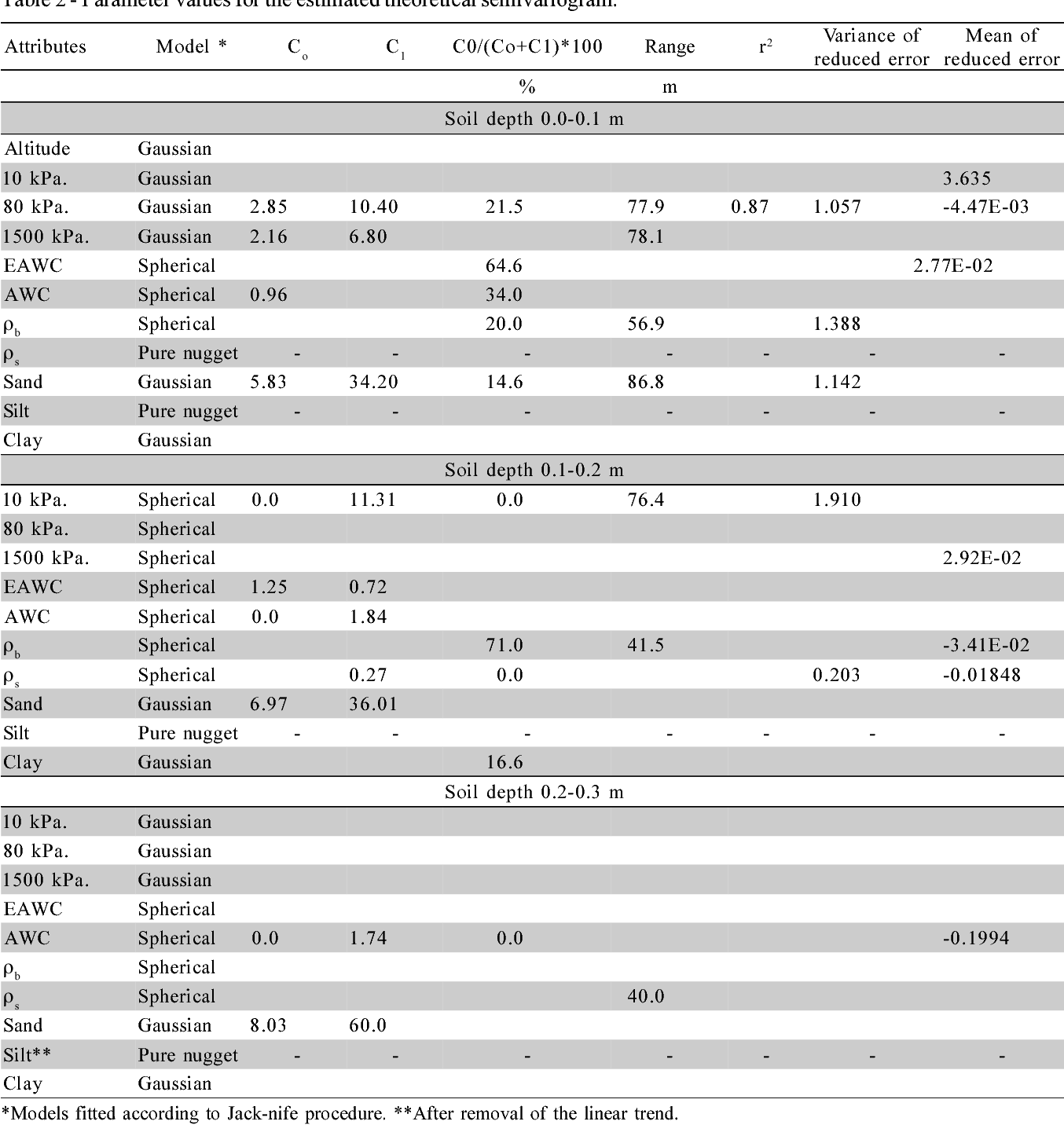•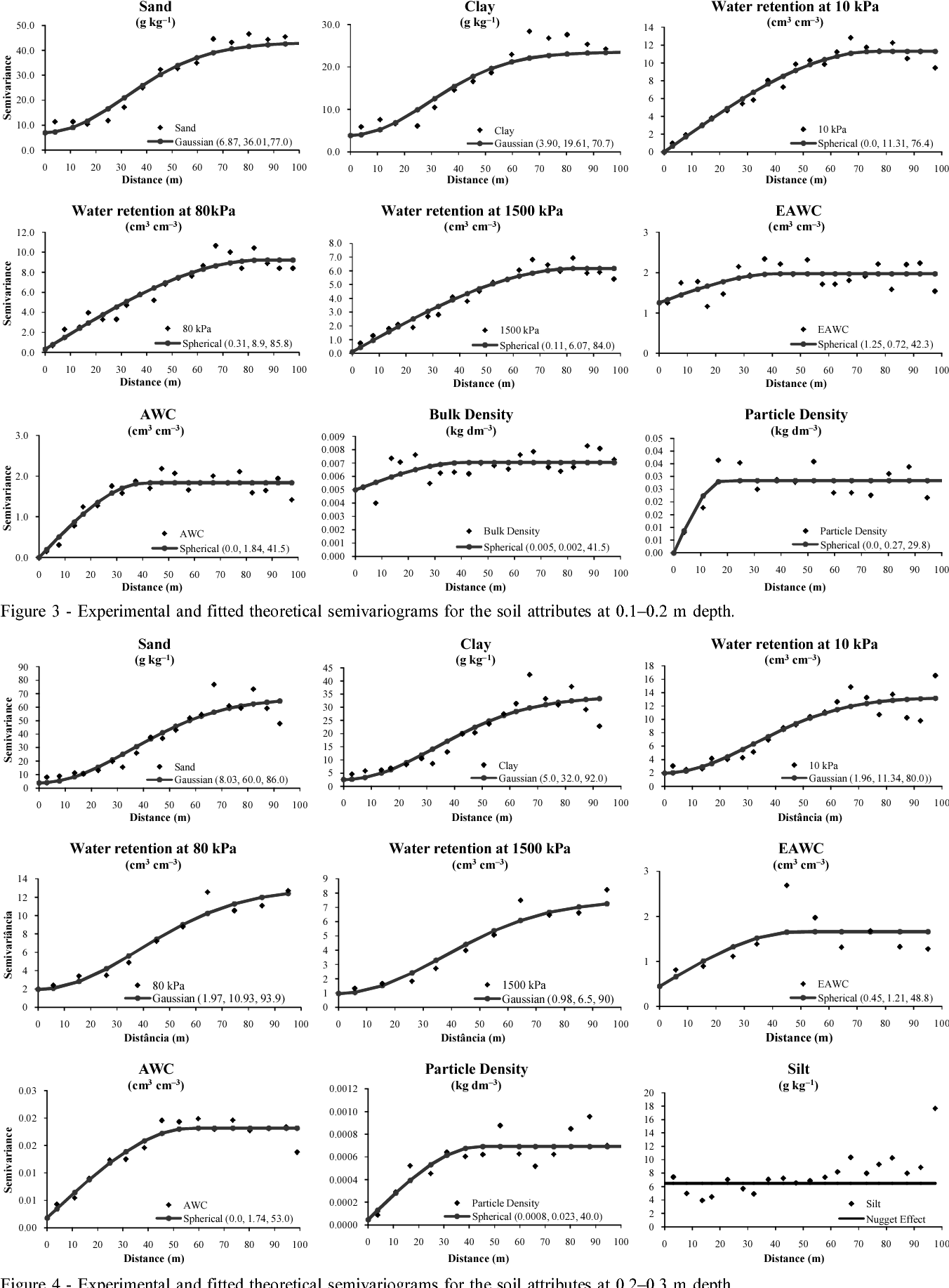Is this relevant?
2002
2002
Fundamental properties of unstable particles, including mass, width, and partial widths, are examined on the basis of the Nielsen… Expand
Is this relevant?
1999
1999
Abstract We study a leptogenesis via decays of heavy Majorana neutrinos produced non-thermally in inflaton decays. We find that… Expand
•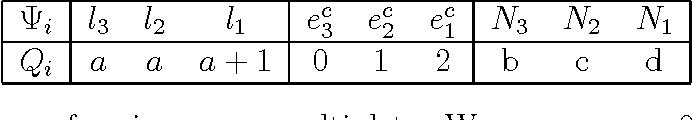Is this relevant?
1998
1998
The index theorem is employed to extend the no-go theorem for lattice chiral Dirac fermions to translation non-invariant and non… Expand
Is this relevant?
1986
1986
Standing stocks and production rates of phytoplankton and planktonic copepods were investigated at 15 stations in the Inland Sea… Expand
•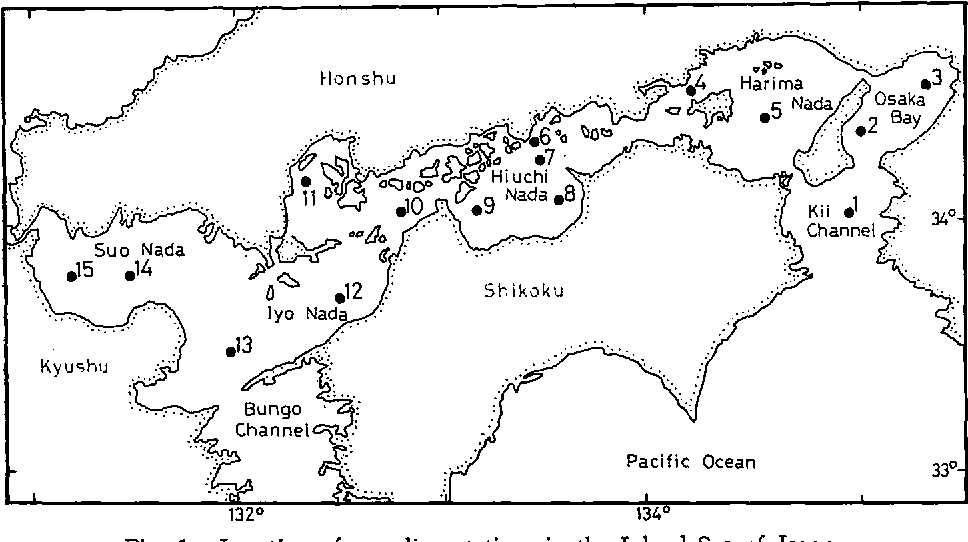Is this relevant?
1982
1982
The Nielsen-Ninomiya theorem asserts the impossibility of constructing lattice models of non-selfinteracting chiral fermions. A… Expand
Is this relevant?